## Machine Learning

Certification Training
17974 Learners
6 Chapters +

# Data Preprocessing - Machine Learning

This is the ‘Data Preprocessing’ tutorial, which is part of the Machine Learning course offered by Simplilearn. We will learn Data Preprocessing, Feature Scaling, and Feature Engineering in detail in this tutorial.

## Objectives

Let’s look at the objectives of Data Preprocessing Tutorial.

• Recognize the importance of data preparation in Machine Learning
• Identify the meaning and aspects of feature engineering
• Standardize data features with feature scaling
• Analyze datasets and its examples
• Explain dimensionality reduction with Principal Component Analysis (PCA)

## Data Preparation in Machine Learning

A quick brief of Data Preparation in Machine Learning is mentioned below.

• Machine Learning depends largely on test data.
• Data preparation is a crucial step to make it suitable for ML.
• A large amount of data is generally required for the most common forms of ML.
• Data preparation involves data selection, filtering, transformation, etc.

## Data Preparation Process

The process of preparing data for Machine Learning algorithm comprises the following:

• Data Selection
• Data Preprocessing
• Data Transformation

### Data Selection

Steps involved in Data Selection involves:

• There is a vast volume, variety, and velocity of available data for a Machine Learning problem.
• This step involves selecting only a subset of the available data.
• The selected sample must be an accurate representation of the entire population.
• Some data can be derived or simulated from the available data if required.
• Data not relevant to the problem at hand can be excluded.

### Data Preprocessing

Let’s understand Data Preprocessing in detail below.

After the data has been selected, it needs to be preprocessed using the given steps:

1. Formatting the data to make it suitable for ML (structured format)
2. Cleaning the data to remove incomplete variables
3. Sampling the data further to reduce running times for algorithms and memory requirements.

Data cleaning at this stage involves filtering it based on the following variables:

Insufficient Data

The amount of data required for ML algorithms can vary from thousands to millions, depending upon the complexity of the problem and the chosen algorithm.

Non-Representative Data

The sample selected must be an exact representation of the entire data, as non-representative data might train an algorithm such that it won't generalize well on new test data.

Substandard Data

Outliers, errors, and noise can be eliminated to get a better fitment of the model. Missing features such as age for 10% of the audience may be ignored completely, or an average value can be assumed for the missing component.

### Data Preprocessing(Contd.)

Selecting the right size of the sample is a key step in data preparation. Samples that are too large or too small might give skewed results.

Sampling Noise

Smaller samples cause sampling noise since they get trained on non-representative data. For example, checking voter sentiment from a very small subset of voters.

Sampling Bias

Larger samples work well as long as there is no sampling bias, that is, hen the right data is picked. For example, sampling bias would occur when checking voter sentiment only for the technically sound subset of voters, while ignoring others.

### Example

Let us look at the Data Sample below: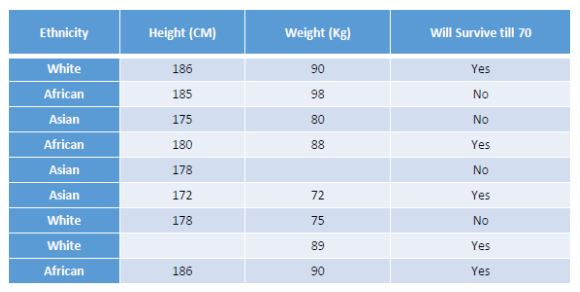Learn in detail about Data Preprocessing. Click here!

### Data Transformation

The selected and preprocessed data is transformed using one or more of the following methods:

1. Scaling: It involves selecting the right feature scaling for the selected and preprocessed data.
2. Aggregation: This is the last step to collate a bunch of data features into a single one.

## Types of Data

Lets us look at the Types of Data below.

### Labeled Data or Training Data

• It is also known as marked (with values) data.
• It assists in learning and forming a predictive hypothesis for future data. It is used to arrive at a formula to predict future behavior.
• Typically 80% of available labeled data is marked for training.

### Unlabeled Data

• Data which is not marked and needs real-time unsupervised learning is categorized as unlabelled data.

### Test Data

• Data provided to test a hypothesis created via prior learning is known as test data.

• Typically 20% of labeled data is reserved for the test.

### Validation data

It is a dataset used to retest the hypothesis (in case the algorithm got overfitted to even the test data due to multiple attempts at testing).

The illustration given below depicts how total available labeled data may be segregated into the training dataset, test dataset, and validation dataset.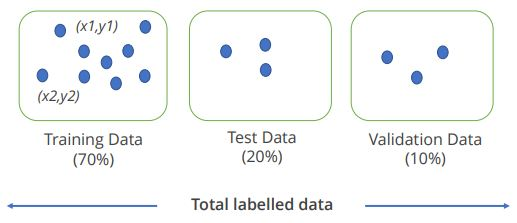## Feature Engineering

The transformation stage in the data preparation process includes an important step known as Feature Engineering.

### Definition of Feature Engineering

Feature Engineering refers to selecting and extracting the right features from the data that are relevant to the task and model in consideration.

## Feature Engineering in ML

The place of feature engineering in the machine learning workflow is shown below: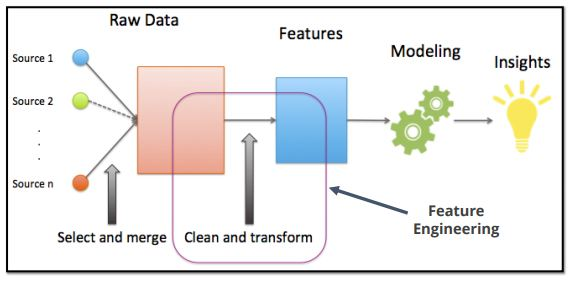## Aspects of Feature Engineering

 Feature Selection Most useful and relevant features are selected from the available data Feature Extraction Existing features are combined to develop more useful ones Feature Addition New features are created by gathering new data Feature Filtering Filter out irrelevant features to make the modeling step easy

## Feature Scaling

Feature scaling is an important step in the data transformation stage of the data preparation process.

### Definition of Feature Scaling

Feature Scaling is a method used in Machine Learning for standardization of independent variables of data features.## Why Feature Scaling?

Let’s understand the importance of Feature Scaling below.

• Let’s consider a situation where input data has two features, one ranging from value 1 to 100 and the other from 1 to 10000.
• This might cause an error in machine learning algorithms, like mean squared error method, when the optimizer tries to minimize larger errors in the second feature.
• The computed Euclidean distances between samples will be dominated by the second feature axis in the K-nearest neighbors (KNN) algorithm.
• The solution lies in scaling all the features on a similar scale (0 to 1) or (1 to 10).

## Techniques of Feature Scaling

There are 2 types of Feature Scaling.

1. Standardization
2. Normalization

### Feature Scaling: Standardization

Let us understand Standardization technique below.

• Standardization is a popular feature scaling method, which gives data the property of a standard normal distribution (also known as Gaussian distribution).
• All features are standardized on the normal distribution (a mathematical model).
• The mean of each feature is centered at zero, and the feature column has a standard deviation of one.

### Standardization: Example

To standardize the jth feature, you need to subtract the sample mean uj from every training sample and divide it by its standard deviation σj as given below: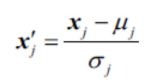Here, xj is a vector consisting of the jth feature values of all training samples n.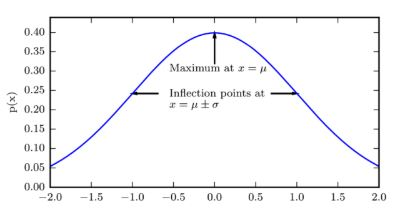Given below is a sample NumPy code that uses NumPy mean and standard

functions to standardize features from a sample data set X (x0, x1...) :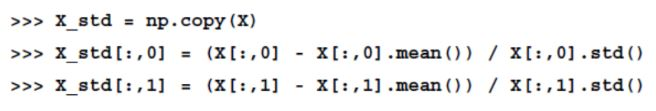The ML library scikit-learn implements a class for standardization called StandardScaler, as demonstrated here: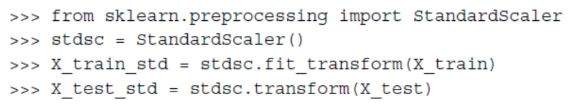### Feature Scaling: Normalization

In most cases, normalization refers to the rescaling of data features between 0 and 1, which is a special case of Min-Max scaling.

### Normalization: Example

In the given equation, subtract the min value for each feature from each feature instance and divide by the spread between max and min.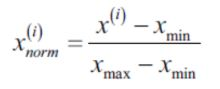In effect, it measures the relative percentage of distance of each instance from the min value for that feature.

The ML library scikit-learn has a MinMaxScaler class for normalization.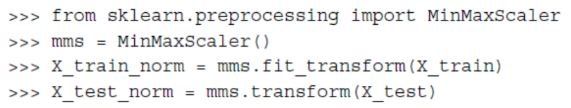## Difference between Standardization and Normalization

The following table shows the difference between standardization and normalization for a sample dataset with values from 1 to 5: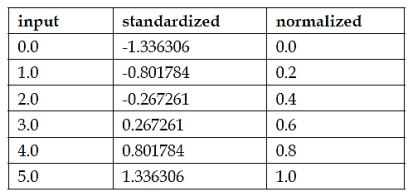## Datasets in Machine Learning

Given below are the Datasets in Machine Learning.

• Machine Learning problems often need training or testing datasets.
• A dataset is a large repository of structured data.
• In many cases, it has input and output labels that assist in Supervised Learning.

### IRIS Dataset

IRIS flower dataset is one of the popular datasets available online and widely used to train or test various ML algorithms.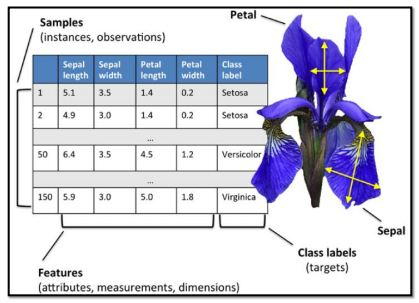### MNIST Dataset

Modified National Institute of Standards and Technology (MNIST) dataset is another popular dataset used in ML algorithms.

• National Institute of Standards and Technology (NIST) is a measurement standards laboratory and a non-regulatory agency of the US Department of Commerce.
• Modified NIST (MNIST) database is a collection of 70,000 handwritten digits and corresponding digital labels
• The digital labels identify each of these digits from 0 to 9.
• It is one of the most common datasets used by ML researchers to test their algorithms.

### Growing Datasets

As the amount of data grows in the world, the size of datasets available for ML development also grows: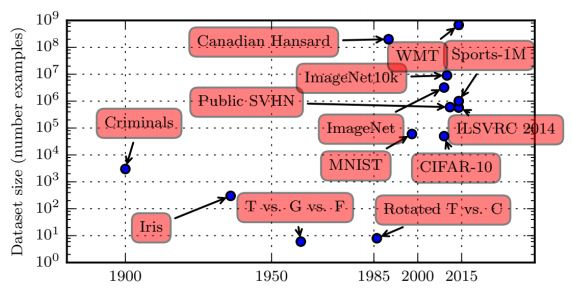## Dimensionality Reduction

Let’s look at some aspects of Dimensionality Reduction below.

• Dimensionality reduction involves the transformation of data to new dimensions in a way that facilitates discarding of some dimensions without losing any key information.
• Large-scale problems bring about several dimensions that can become very difficult to visualize
• Some of such dimensions can be easily dropped for a better visualization.

Example: Car attributes might contain maximum speed in both units, kilometer per hour, and miles per hour. One of these can be safely discarded in order to reduce the dimensions and simplify the data.

## Dimensionality Reduction with Principal Component Analysis

Below mentioned are some of the Dimensionality Reduction aspects.

• Principal component analysis (PCA) is a technique for dimensionality reduction that helps in arriving at better visualization models.
• Let’s consider the pilots who like to fly radio-controlled helicopters. Assume x1 = the piloting skill of the pilot and x2 = passion to fly.
• RC helicopters are difficult to fly and only those students that truly enjoy flying can become good pilots. So, the two factors x1 and x2 are correlated, and this correlation may be represented by the piloting “karma” u1 and only a small amount of noise lies off this axis (represented by u2 ).
• Most of the data lie along u1, making it the principal component.
• Hence, you can safely work with u1 alone and discard u2 dimension. So, the 2D problem now becomes a 1D problem.

Keen on learning Machine Learning? Click for course description!

## Principal Component Analysis (PCA)

Let’s look at some aspects of Principal Component Analysis below.

• Before the PCA algorithm is developed, you need to preprocess the data to normalize its mean and variance.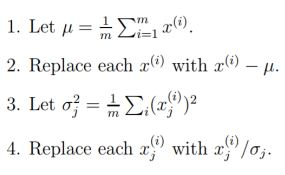• Steps 1 and 2 reduce the mean of the data, and steps 3 and 4 rescale each coordinate to have unit variance. It ensures that different attributes are treated on the same scale.
• For instance, if x1 was maxed speed in mph (taking values in high tens or low hundreds) and x2 was the number of seats (taking values 2-4), then this renormalization rescales the attributes to make them more comparable to each other.

## Principal Component Analysis (PCA)(Contd.)

How do you find the axis of variation u on which most of the data lies?

• When you project this data to lie along the axis of the unit vector, you would like to preserve most of it, such that its variance is maximized (which means most data is covered).
• Intuitively, the data starts off with some amount of variance (information).
• The figure shows this normalized data.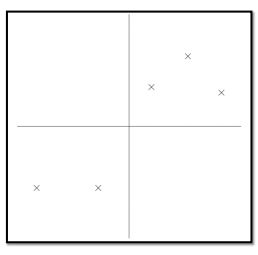• Let’s project data onto different u axes as shown in the charts given on the left.
• Dots represent the projection of data points on this line.
• In figure A, projected data has a large amount of variance, and the points are far from zero.
• In figure B, projected data has a low amount of variance, and the points are closer to zero.
• Hence, figure A is a better choice to project the data.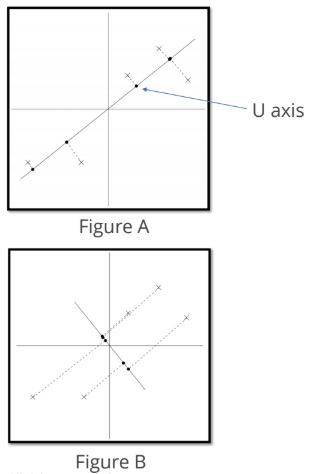• The length of projection of x on a unit vector u is given by xTu. This also represent the distance of the projection of x from the origin.
• Hence, to maximize the variance of the projections, you can choose a unit length u: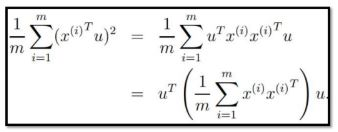• You get the principal Eigenvector* of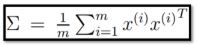• It is also known as the covariance matrix of the data (assuming that it has zero mean).
• Generally, if you need to project data onto the k-dimensional subspace (k < n), you choose u1, u2...uk to be the top k Eigenvectors of ∑.
• All the ui now form a new orthogonal basis for the data.
• Then, to represent x(i) in this new basis, you need to compute the corresponding vector: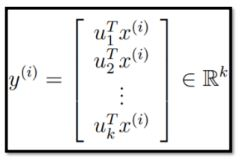• The vector y(i) is a lower k-dimensional approximation of x(i). This is known as the dimensionality reduction.
• The vectors u1,u2...uk are called the first k principal components of the data.

## Applications of PCA

Given below are the application of PCA.

### Noise Reduction

PCA can eliminate noise or noncritical aspects of the data set to reduce complexity. Also, during image processing or comparison, image compression can be done with PCA, eliminating the noise such as lighting variations in face images.

### Compression

It is used to map high dimensional data to lower dimensions. For example, instead of having to deal with multiple car types (dimensions), we can cluster them into fewer types.

### Preprocess

It reduces data dimensions before running a supervised learning program and saves on computations as well as reduces overfitting.

## PCA: 3D to 2D Conversion

3D Data ----changes to----- After PCA, one finds only two dimensions being important—Red and Green that carry most of the variance. The blue dimension has limited variance, and hence it is eliminated.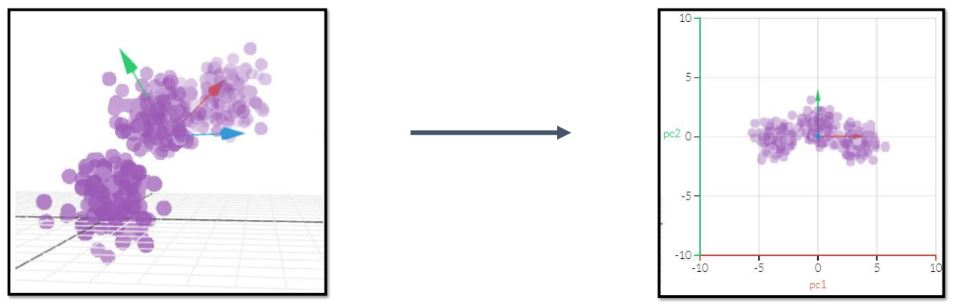## Key Takeaways

Let us go through what you have learned so far in this Data Preprocessing tutorial.

• Data preparation allows simplification of data to make it ready for Machine Learning and involves data selection, filtering, and transformation.
• Data must be sufficient, representative of real-world data, and of high quality.
• Feature Engineering helps in selecting the right features and extracting the most relevant features.
• Feature scaling transforms features to bring them on a similar scale, in order to make them comparable in ML routines.
• Dimensionality Reduction allows reducing dimensions in datasets to simplify ML training.

## Conclusion

This concludes “Data Preprocessing” tutorial. In the next lesson, we will learn "Math Refresher"

### Find our Machine Learning Online Classroom training classes in top cities:

Name Date Place
Machine Learning 24 Apr -29 May 2021, Weekend batch Your City View Details
Machine Learning 3 May -21 May 2021, Weekdays batch San Francisco View Details
Machine Learning 7 May -11 Jun 2021, Weekdays batch New York City View Details
Related Courses
Learner Reviews
Related Articles
• Disclaimer
• PMP, PMI, PMBOK, CAPM, PgMP, PfMP, ACP, PBA, RMP, SP, and OPM3 are registered marks of the Project Management Institute, Inc.

#### Request more information

###### For business
Name*
Email*
Phone Number*
Your Message (Optional)
###### Our consultants will get in touch with you soon.

A Simplilearn representative will get back to you in one business day.

First Name*
Last Name*
Work Email*
Phone Number*
Company*
Job Title*